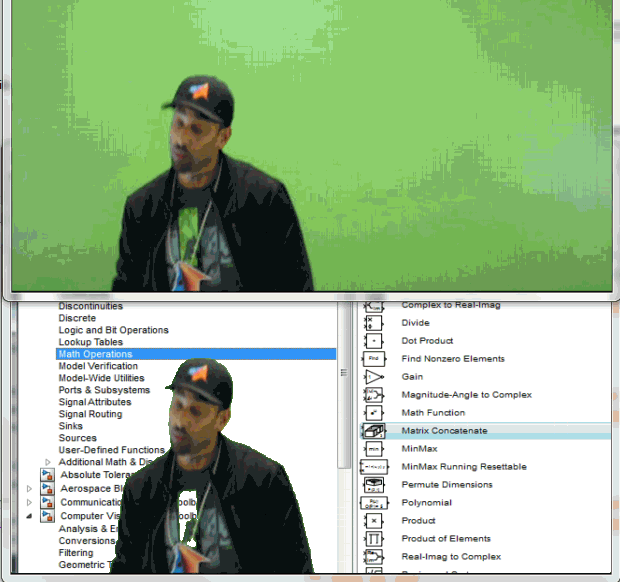# A MATLAB vs. Simulink battle? I’m in!

When I saw the latest post by Matt Tearle on Steve's blog, I had to do something.

Since the video at the center of this post is about a rap battle between MATLAB and Simulink, I thought it would be interesting to bring that to a higher level and see how the video processing could have been implemented in Simulink instead of MATLAB.

Seriously... who wants to write code when you can simply connect blocks?

Overview

Since I have absolutely zero knowledge in image processing, I simply re-implemented the algorithm suggested by Matt in Simulink. The resulting model looks like: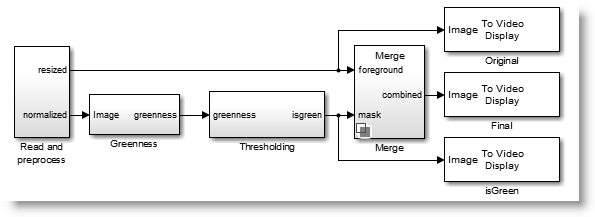Let's look at the details.

Importing the video

To begin, I used the From Multimedia File from the DSP System Toolbox to directly read the AVI-file with our rappers in front of a green background. Then I use the resize block from the Computer Vision System Toolbox. This allows me to work on images of a relatively small size. After, I normalize the data, as suggested by Matt.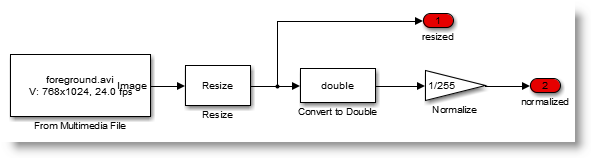The Greenness factor

I really like the simple equation the Matt figured out to calculate the "greenness" of each pixel in the image. In MATLAB, it looks like:

% Greenness = G*(G-R)*(G-B) greenness = yd(:,:,2).*(yd(:,:,2)-yd(:,:,1)).*(yd(:,:,2)-yd(:,:,3));

In Simulink, I can use Selector blocks to separate the three colors (third dimension of the image signal) and implement Matt's equation: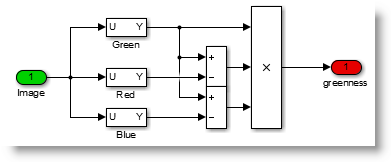Thresholding

The next piece of code is something that many users struggle to figure out:

thresh = 0.3*mean(greenness(greenness>0));

To implement logical indexing (e.g. A(A>0)) in Simulink, we need to combine the Find block with a Selector block. This results in a variable-size signal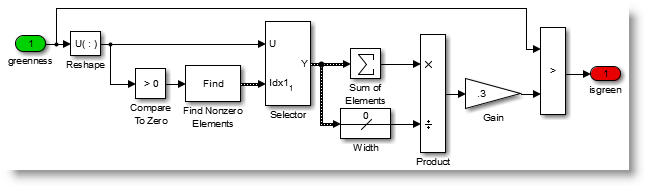Combining the images

The last line we need to implement is to replace the pixels identified as green in the foreground image but their values in the background image. In MATLAB, this is accomplished using this line:

rgb2(isgreen) = rgb1(isgreen);

In Simulink, we need to extend the logic seen at the previous step. We first select the desired pixels from the background image, and then assign them to the foreground image: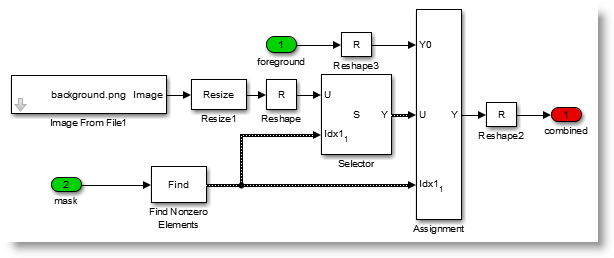Note that the Computer Vision System Toolbox has a Compositing block implementing this functionality.

Here is what the final result looks like: